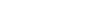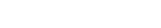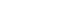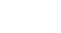Null hypothesis: is the claim being assessed in a hypothesis.  For a claim to be a testable null hypothesis, it must specify a value for some population parameter that can form the basis for assuming a sampling distribution for a test statistic.

Alternative hypothesis: The alternative hypothesis proposes what we should conclude if we find the null hypothesis to be unlikely.

Two-sided alternative: An alternative hypothesis is two-sidedwhen we are interested in deviations in either direction away from the hypothesized parameter value.

One-sided alternative: An alternative hypothesis is one-sided when we are interested in deviations in only one direction away from the hypothesized parameter value. The form for that isP-value: The probability of observing a value for a test statistic at least as far from the hypothesized value as the statistic value actually observed if the null hypothesis is true. A small P-value indicates either that the observation is improbable or that the probability calculation was based on incorrect assumptions. The assumed truth of the null hypothesis is the assumption under suspicion.

One-proportion z-test: A test of the null hypothesis that the proportion of a single sample equals a specified valueby referring the statisticto a Standard Normal model.

Applying knowledge:

We start with a null hypothesis specifying the parameter of a model we’ll test using our data.

Then we also have to consider our alternative hypothesis. Our alternative hypothesis can be one- or two-sided, depending on what we want to learn.

We must check the appropriate assumptions and conditions before proceeding with our test.

If the data are out of line with the null hypothesis model, the P-value will be small and we will reject the null hypothesis.

If the data are consistent with the null hypothesis model, the P-value will be large and we will not reject the null hypothesis.

We must always state our conclusion in the context of the original question.

Most importantly, the confidence intervals and hypothesis tests go hand in hand in helping us think about models. A hypothesis test makes a yes/no decision about the plausibility of a parameter value. The confidence interval shows us the range of plausible values for the parameter.

Cite this article as: William Anderson (Schoolworkhelper Editorial Team), "Null hypothesis, Alternative hypothesis, P value and Z test," in SchoolWorkHelper, 2019, https://schoolworkhelper.net/null-hypothesis-alternative-hypothesis-p-value-and-z-test/.